Published © GPL3+

# Building a Custom JetBot with Jetson Nano

Take Nvidia's JetBot platform to the next level by expanding the functionality of the Jetbot API by adding new sensors and drivers.

IntermediateFull instructions provided5 hours9,482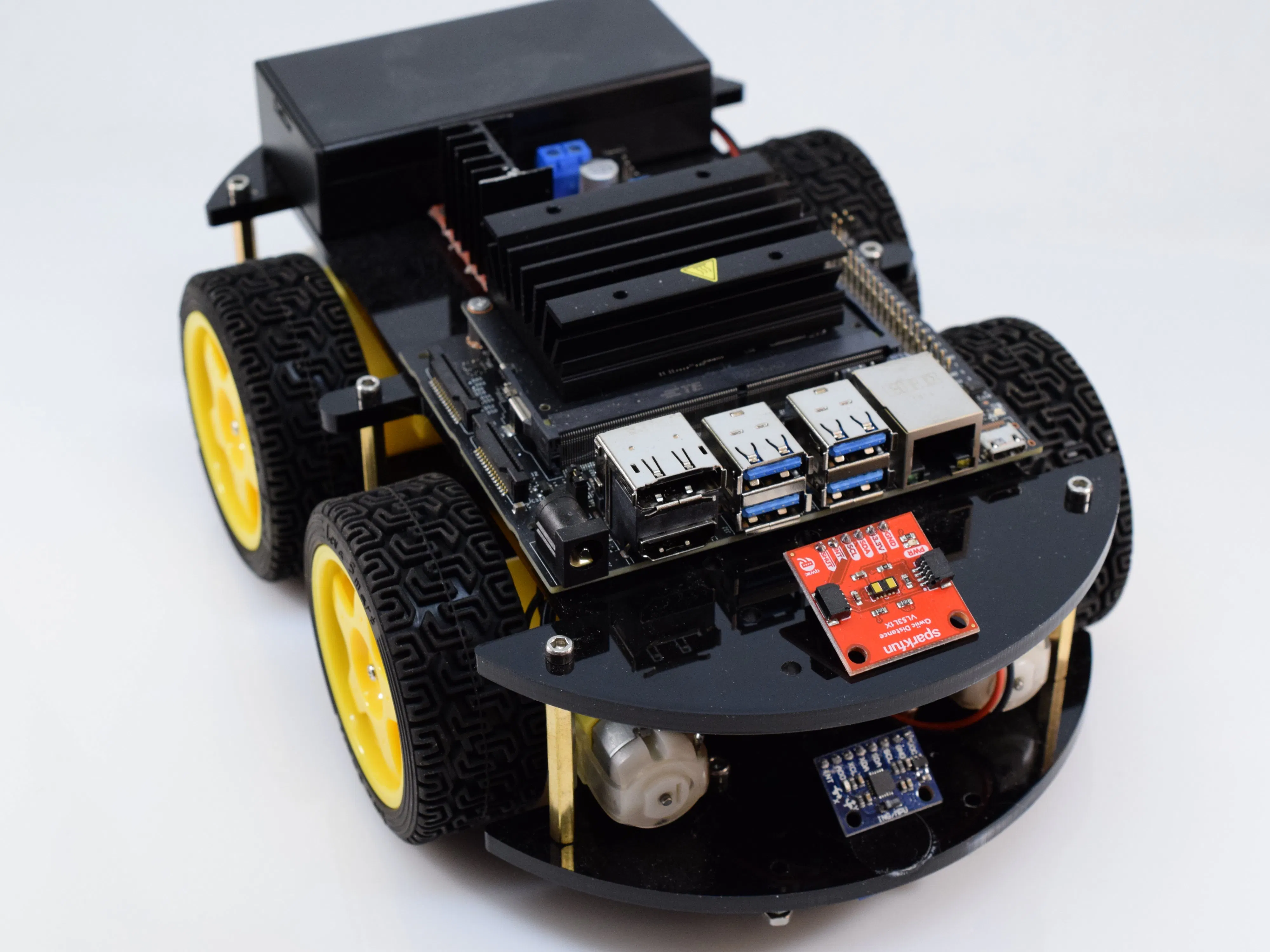## Things used in this project

### Hardware components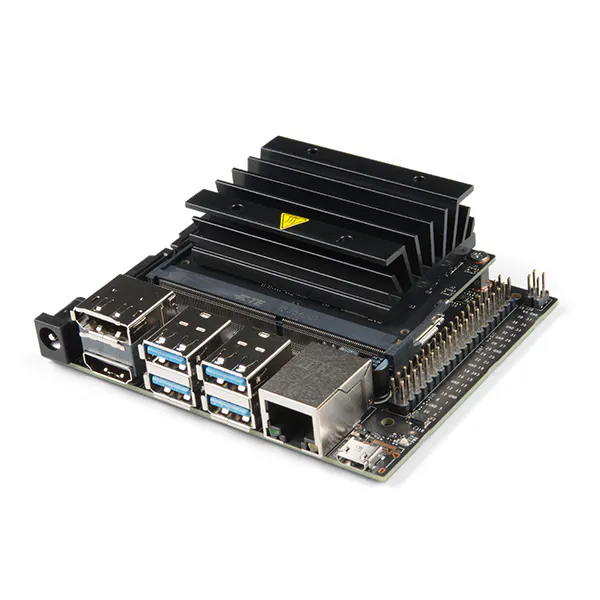NVIDIA Jetson Nano Developer Kit
×1
 DC motor (generic)
×2Texas Instruments Dual H-Bridge motor drivers L293D
×1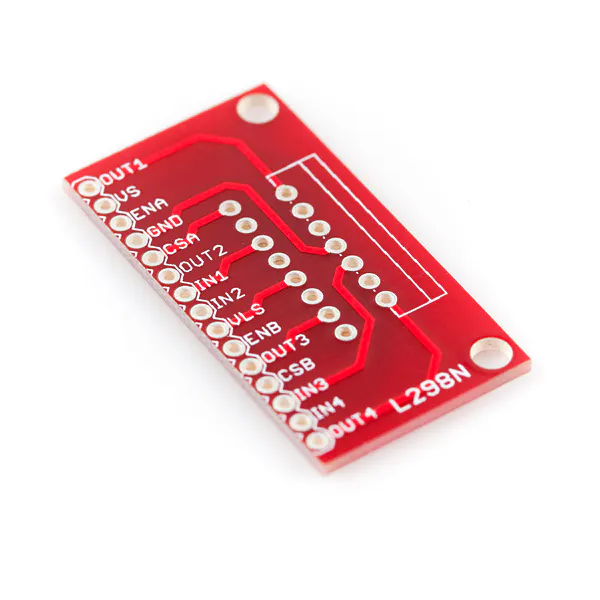SparkFun Full-Bridge Motor Driver Breakout - L298N
×1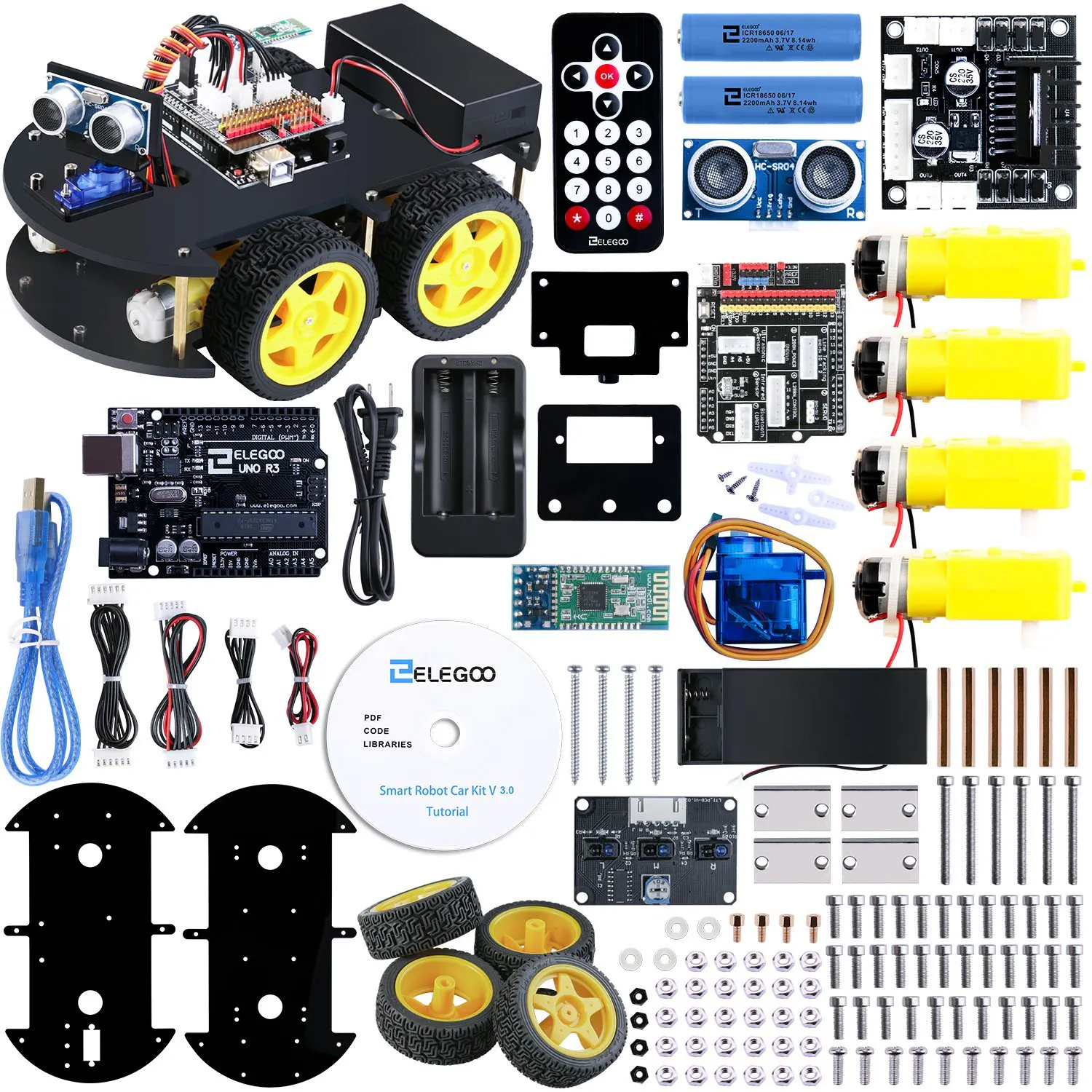ELEGOO EL-KIT-012 UNO Project Smart Robot Car Kit V 3.0
×1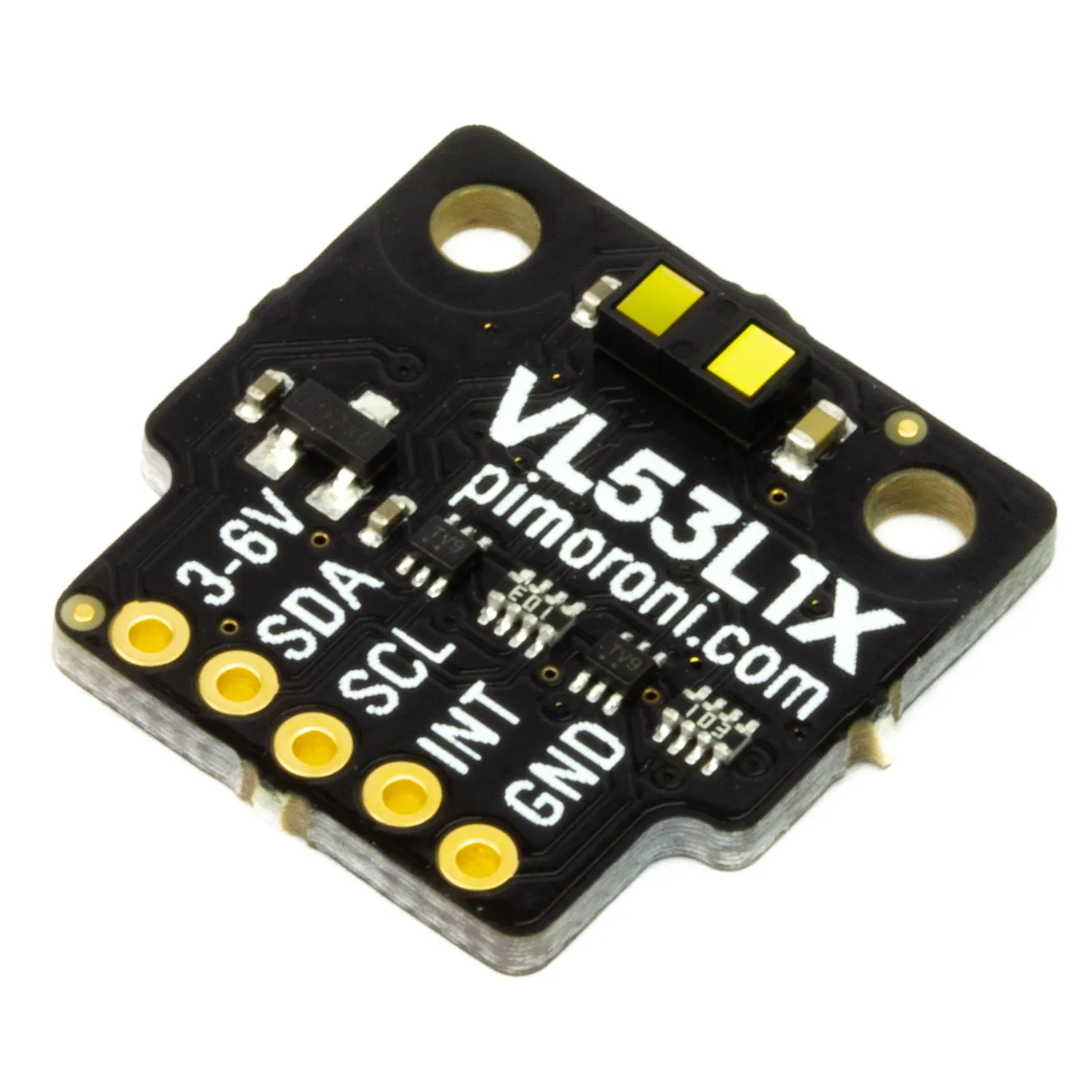Pimoroni VL53L1X Time of Flight (ToF) Sensor Breakout
×1

### Software apps and online services

 Python 3Jupyter Notebook
 Jetbot

### Hand tools and fabrication machines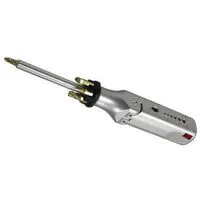Multitool, ScrewdriverSoldering iron (generic)

Read more

## Schematics

### GPIO Header Pinout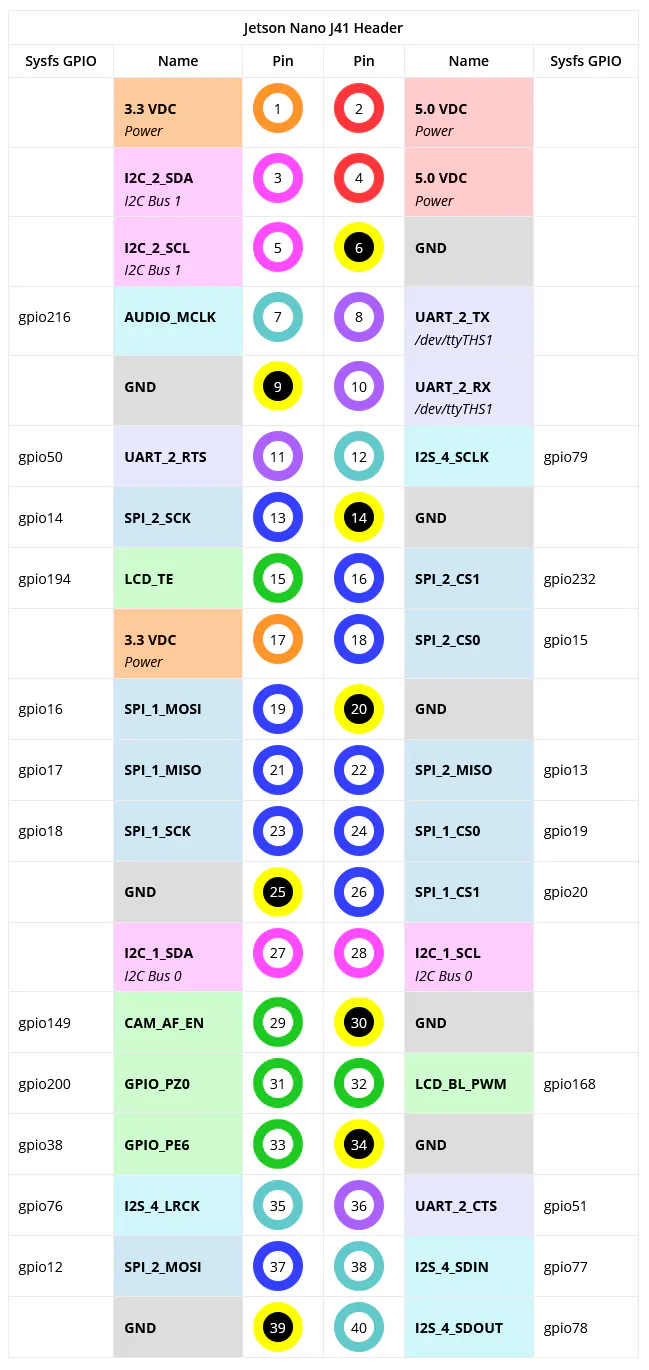## Code

### Modified robot.py

Python
```import time
import traitlets
from traitlets.config.configurable import SingletonConfigurable
from .motor import Motor
from .vl53l1x import Vl53l1x
import RPi.GPIO as GPIO

class Robot(SingletonConfigurable):

left_motor = traitlets.Instance(Motor)
right_motor = traitlets.Instance(Motor)
tof_sensor = traitlets.Instance(Vl53l1x)

distance = traitlets.Integer(default_value=1).tag(config=True)

# config
i2c_bus = traitlets.Integer(default_value=1).tag(config=True)
left_motor_alpha = traitlets.Float(default_value=1.0).tag(config=True)
right_motor_alpha = traitlets.Float(default_value=1.0).tag(config=True)

GPIO.setmode(GPIO.BOARD)

def __init__(self, *args, **kwargs):
super(Robot, self).__init__(*args, **kwargs)
self.left_motor = Motor(35, 36, freq=50, alpha=self.left_motor_alpha)
self.right_motor = Motor(37, 38, freq=50, alpha=self.right_motor_alpha)
self.tof_sensor = Vl53l1x(self, i2c_bus_num=self.i2c_bus, i2c_addr=0x29)

@observe('distance')
def _observe_distance(self, change):
new_distance_value = change['new']

def set_motors(self, left_speed, right_speed):
self.left_motor.value = left_speed
self.right_motor.value = right_speed

def forward(self, speed=1.0, duration=None):
self.left_motor.value = speed
self.right_motor.value = speed

def backward(self, speed=1.0):
self.left_motor.value = -speed
self.right_motor.value = -speed

def left(self, speed=1.0):
self.left_motor.value = -speed
self.right_motor.value = speed

def right(self, speed=1.0):
self.left_motor.value = speed
self.right_motor.value = -speed

def stop(self):
self.left_motor.value = 0
self.right_motor.value = 0
```

### Modified motor.py

Python
```import atexit
import traitlets
from traitlets.config.configurable import Configurable
import RPi.GPIO as GPIO

class Motor(Configurable):

value = traitlets.Float()

# config
alpha = traitlets.Float(default_value=1.0).tag(config=True)
beta = traitlets.Float(default_value=0.0).tag(config=True)

def __init__(self, pin1, pin2, freq=50, *args, **kwargs):
super(Motor, self).__init__(*args, **kwargs)  # initializes traitlets
self.pins = {'out1' : pin1, 'out2' : pin2}
self.PWMs = []
atexit.register(self._release)
for out, pin in self.pins.items():
GPIO.setup(pin, GPIO.OUT, initial=GPIO.HIGH)
self.PWMs.append(GPIO.PWM(pin, freq))
self.PWMs.start(0)
self.PWMs.start(0)

@traitlets.observe('value')
def _observe_value(self, change):
self._write_value(change['new'])

def _write_value(self, value):
"""Sets motor value between [-1, 1]"""
mapped_value = int(100.0 * (self.alpha * value + self.beta))
speed = min(max(abs(mapped_value), 0), 100)
self.PWMs.changeDutyCycle(speed)
self.PWMs.changeDutyCycle(speed)
if mapped_value < 0:
self.PWMs.changeDutyCycle(0)
else:
self.PWMs.changeDutyCycle(0)

def _release(self):
"""Stops motor by releasing control"""
self.PWMs.stop()
self.PWMs.stop()
```

### New vl53l1x.py file

Python
```import atexit
import traitlets
from traitlets.config.configurable import Configurable
import concurrent.futures
import VL53L1X
import Robot

class Vl53l1x(Configurable):

distance = traitlets.Integer()

def __init__(self, robot_instance, i2c_bus_num=1, i2c_addr=0x29, *args, **kwargs):
self.tof = VL53L1X.VL53L1X(i2c_bus=i2c_bus_num, i2c_address=i2c_addr)
self.tof.open()
self.SHORT = 1
self.MEDIUM = 2
self.LONG = 3
self.robot = robot_instance

def read_distance(self, mode=1):
self.tof.start_ranging(mode)
with concurrent.futures.ThreadPoolExecutor() as executor:
future = executor.submit(_get_distance, self.tof)
val = future.result()
self.robot.distance = val

def _get_distance(tof_sensor):
return tof_sensor.get_distance()
```

## Credits

### Arduino “having11” Guy

102 projects • 733 followers
19 year-old IoT and embedded systems enthusiast. Also produce content for Hackster.io and love working on projects and sharing knowledge.